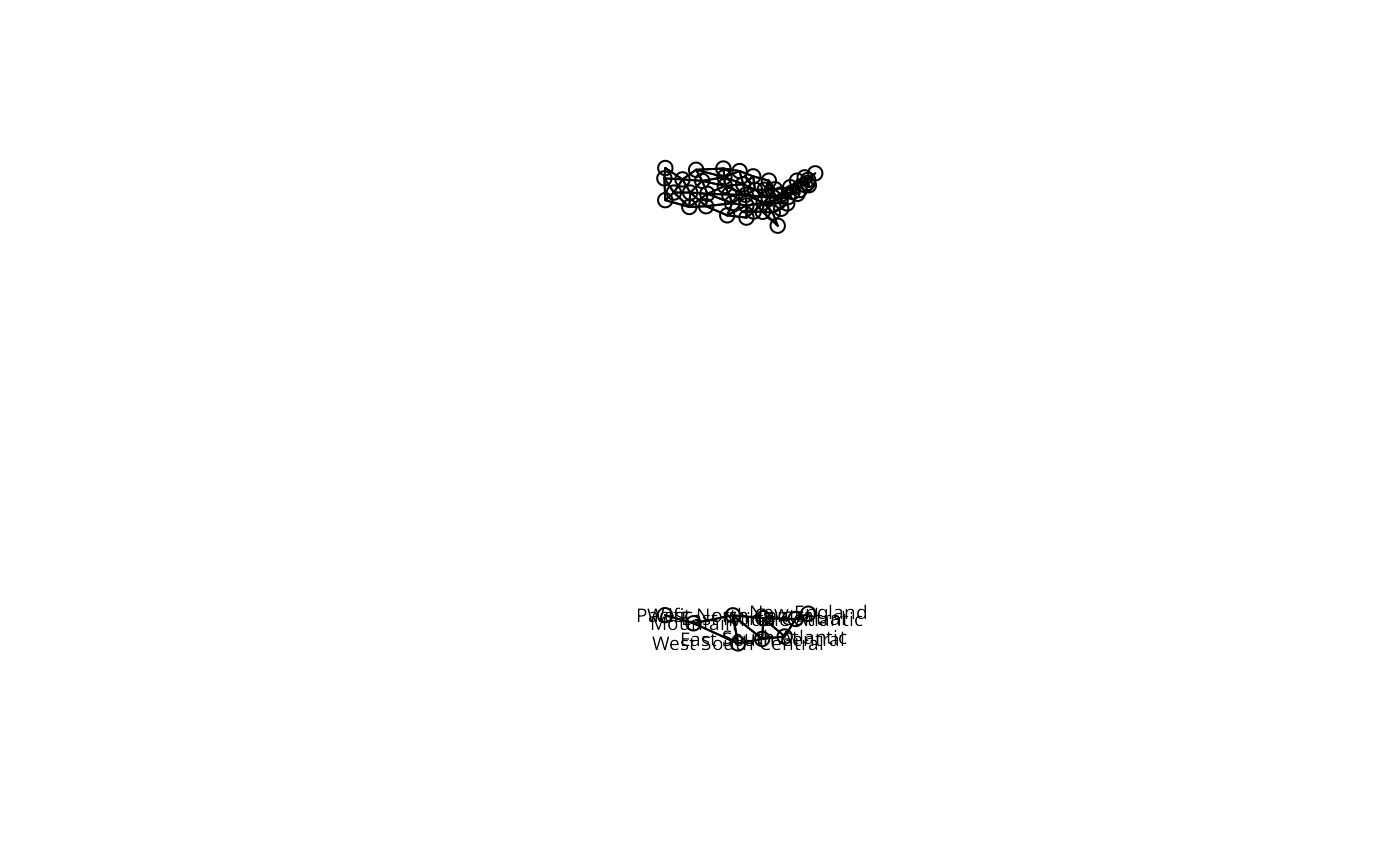The method aggregates a spatial neighbours object, creating a new object listing the neighbours of the aggregates.

# S3 method for nb
aggregate(x, IDs, remove.self = TRUE, ...)

## Arguments

x

an nb neighbour object

IDs

a character vector of IDs grouping the members of the neighbour object

remove.self

default TRUE: remove self-neighbours resulting from aggregation

...

unused - arguments passed through

## Value

an nb neighbour object, with empty aggregates dropped.

## Author

Roger Bivand Roger.Bivand@nhh.no

## Note

Method suggested by Roberto Patuelli

## Examples

data(used.cars, package="spData")
data(state)
cont_st <- match(attr(usa48.nb, "region.id"), state.abb)
cents <- as.matrix(as.data.frame(state.center))[cont_st,]
opar <- par(mfrow=c(2,1))
plot(usa48.nb, cents, xlim=c(-125, -65), ylim=c(25, 50))
IDs <- as.character(state.division[cont_st])
agg_cents <- aggregate(cents, list(IDs), mean)
agg_nb <- aggregate(usa48.nb, IDs)
plot(agg_nb, agg_cents[, 2:3], xlim=c(-125, -65), ylim=c(25, 50))
text(agg_cents[, 2:3], agg_cents[, 1], cex=0.6)par(opar)